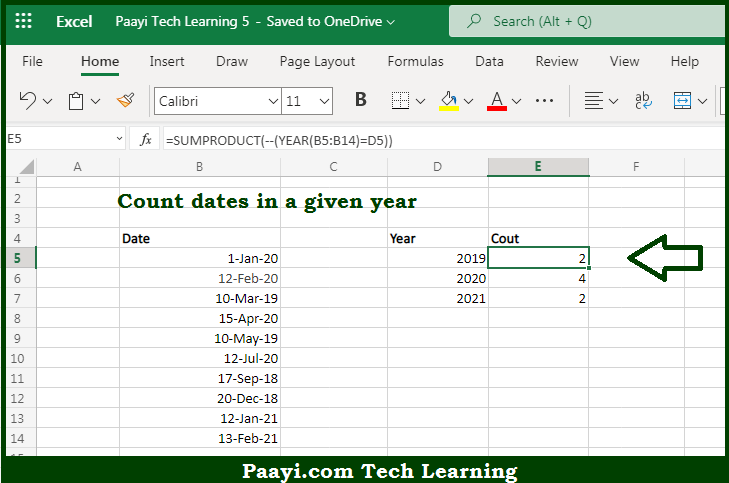# Learn How to Count Dates in Given Year in Microsoft Excel

Written by | 0 Comments | 583 Views

In this article, you will learn how to COUNT various things in Microsoft Excel using a single or combination of functions and its purpose in Microsoft Excel. You will also get to know how to count dates in a given year and see the generic formula

Count Dates in Given Year in Microsoft Excel

The main purpose of this formula is to count dates in a given year. Here we will learn how to count the dates of the year provided in Microsoft Excel. That implies, with the help of a formula based on the SUMPRODUCT and YEAR functions you can able to count dates in the given year. So, with the help of this formula, you can able to count the range of cells having dates by the given year.

General Formula to Count Dates in Given Year

=SUMPRODUCT(--(YEAR(dates)=year))

The Explanation for the Count Dates in Given YearSo we know that with the help of the given formula above you can able to count dates in a given year. Here we will learn how to count the dates of the year provided in Microsoft Excel. As we know that, The YEAR function is issued to extract the year from a valid date. That is why we are using the YEAR function and array of dates in the named range "dates", so we get back an array of results. Each date is compared to the year value is provided in the given column, to produce an array of TRUE FALSE values. So, with the help of this formula, you can able to count dates in a given year.